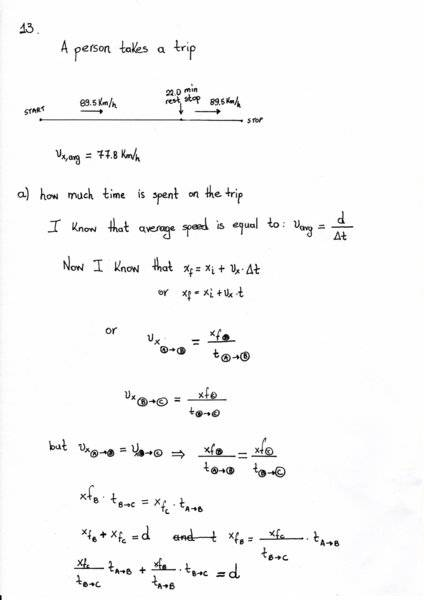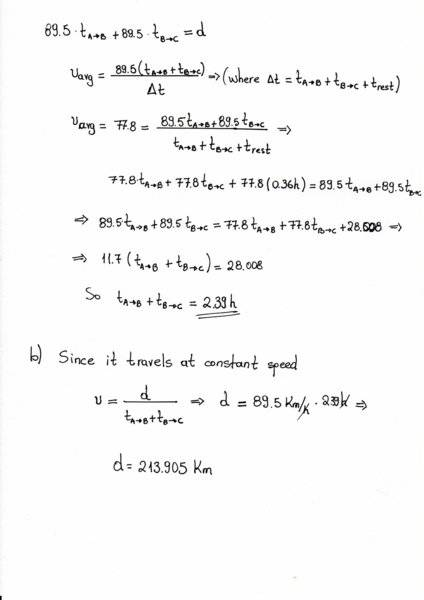# A person takes a trip: Find the time spent on the trip, etc.

• Alexanddros81
I get 473.68 km for the distance traveled. I didn't check your calculation, but your answer is right.In summary, a person takes a trip with an average speed of 77.8 Km/h, driving with a constant speed of 89.5 Km/h except for a 22.0-min rest stop. The time spent on the trip is not affected by the rest stop and can be calculated using the equation v_avg = D/t. Therefore, using this equation and the given information, the total distance traveled by the person is 473.68 km.

## Homework Statement

A person takes a trip, driving with a constant of 89.5 Km/h, except for a 22.0-min rest stop.
If the person's average speed is 77.8 Km/h,
(a) how much time is spent on the trip and
(b) how far does the person travel?

This is problem 13, Chapter 2 from Serway Physics for scientists and engineers Volume 1 9th edition

## The Attempt at a Solution[/B]
Is my solution correct?

#### Attachments

When you wrote $$V_{avg} = {89.5 t_{a \to b} + 89.5 t_{b \to c} \over t_{a \to b} + t_{b \to c} + t_{rest}}$$, that was correct but I think they mean to include the rest stop in the time taken.

I disagree with your numbers. It makes no sense to to split the time interval into 3 parts because it doesn't matter when the driver rests. For all you know, the driver can start 22.0 min late and have no rest stop and it wouldn't make a difference to the average speed and the distance traveled. The average speed is $$v_{avg}=\frac{D}{t_{rest}+t_{drive}}$$where ##D## is the total distance traveled. You also have a second equation relating ##D## and ##t_{drive}##. The two equations comprise a system of two equations and two unknowns, ##D## and ##t_{drive}##, that you have to solve.

•Alexanddros81
Yes, your solution is correct with the small correction that 77.8 * (22/60) = 28.53 not 28.008. That’s just some sort of round off error, but I can’t spot where or why it is so big.

I think you could do this much more succinctly effectively jumping right to the middle of your calculation by appropriate logic. What you arrive at third line from the bottom of part a:

(tdrive+ 22 min) * vavg = tdrive * vdrive

seems readily apparent and could easily have been written as the starting point of the solution.

•Alexanddros81
•